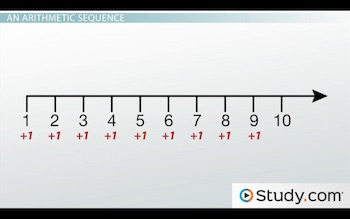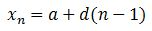# How and Why to Use the General Term of an Arithmetic Sequence

An error occurred trying to load this video.

Try refreshing the page, or contact customer support.

Coming up next: The Sum of the First n Terms of an Arithmetic Sequence

### You're on a roll. Keep up the good work!

Replay
Your next lesson will play in 10 seconds
• 0:01 An Arithmetic Sequence
• 1:23 The General Term
• 2:04 Using the General Term
• 3:26 Why We Use the General Term
• 4:08 Lesson Summary
Save Save

Want to watch this again later?

Timeline
Autoplay
Autoplay
Speed Speed Audio mode

#### Recommended Lessons and Courses for You

Lesson Transcript
Instructor: Yuanxin (Amy) Yang Alcocer

Amy has a master's degree in secondary education and has taught math at a public charter high school.

Watch this video lesson to learn the formula for the general term of an arithmetic sequence. Also learn why this formula makes working with an arithmetic sequence much easier.

## An Arithmetic Sequence

We will be talking about the general term of an arithmetic sequence in this video lesson. You will see the formula we use for the general term of any arithmetic sequence and how easy it is to use it.

We begin with our arithmetic sequence, which is a string of numbers where each number is the previous number plus a constant. So, if you look at our number line, you will see that it is an arithmetic sequence since each number is the previous number plus 1.This difference between the numbers is called the common difference. We write our sequences inside curly brackets with commas in between the numbers.

Another way of thinking about arithmetic sequences is to think of things that grow over time. For example, we can represent the number of leaves a peach tree has each day with an arithmetic sequence. If our peach tree begins with 10 leaves and grows 15 new leaves each day, we can write the arithmetic sequence {10, 25, 40, . . .} to show the number of leaves that our peach tree has each day. This sequence ends when our peach tree stops growing.

So, while some arithmetic sequences have an end, like our peach tree sequence, some don't and continue on forever, like our number line. Because our arithmetic sequences have a pattern, we have a formula for the general term of an arithmetic sequence.

## The General Term

This general term is the formula that is used to calculate any number in an arithmetic sequence.The formula tells us that if we wanted to find a particular number in our sequence, x sub n, we would take our beginning number, a, and add our common difference, d, times n minus 1, which is the location of our desired number minus 1. If we are looking for the 30th number, our n is 30, so our formula begins with x sub 30, and n - 1 equals 29 (30 - 1).

## Using the General Term

Let's look at an example to see how we use this general term. Here's a new sequence: {2, 4, 6, . . .}. We want to find the value of the number that is the 25th place in our sequence. Our n in this case is 25. Before we go further, we first have to check whether this sequence is an arithmetic sequence or not.

To do that, we look at the difference between each successive pair of numbers to see if this difference is the same. If it is, then we are looking at an arithmetic sequence. If it isn't, then we can't use our general term formula to find our answer. In our case, we subtract 4 - 2 and also 6 - 4 to see if they equal the same thing. They do; they both equal 2. So, this means the sequence is an arithmetic sequence; it is an arithmetic sequence that jumps by 2 with every number.

To unlock this lesson you must be a Study.com Member.

### Register to view this lesson

Are you a student or a teacher?

#### See for yourself why 30 million people use Study.com

##### Become a Study.com member and start learning now.
Back
What teachers are saying about Study.com

### Earning College Credit

Did you know… We have over 200 college courses that prepare you to earn credit by exam that is accepted by over 1,500 colleges and universities. You can test out of the first two years of college and save thousands off your degree. Anyone can earn credit-by-exam regardless of age or education level.